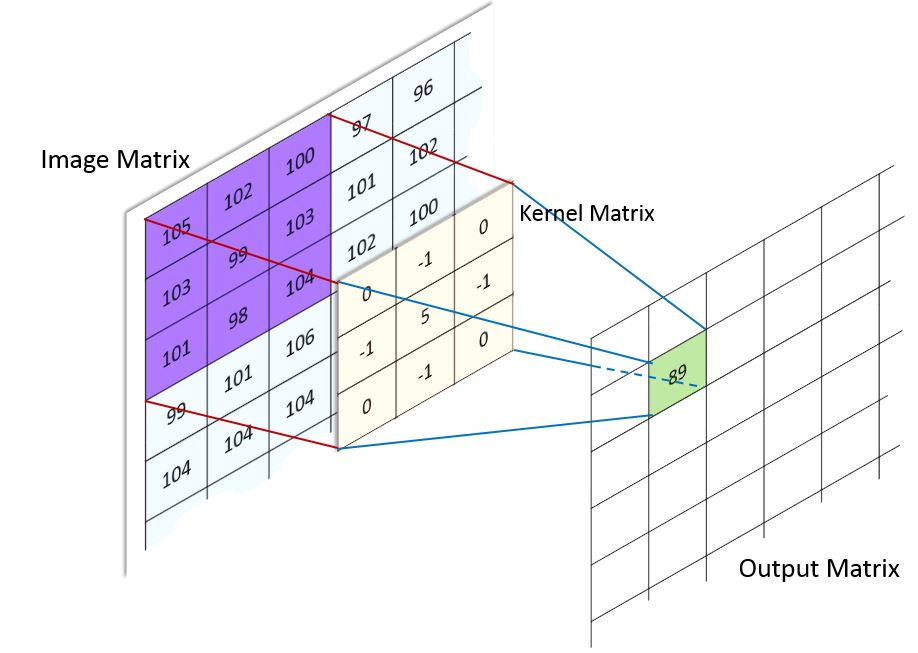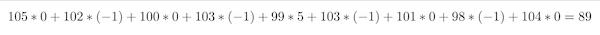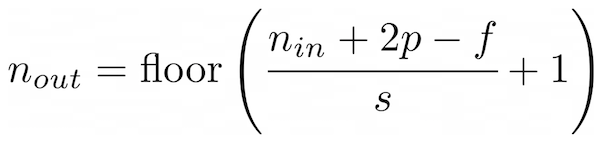###Bite 331. Convolution in Neural Networks

Finally a Bite about deep learning! At least, about one of the major building blocks of modern deep learning architectures: the Convolutional Neural Network (CNN or convnet for short).

The main idea of the convolutional layer in a convnet is to apply a filter, also called kernel, to a certain area of the input image. This will produce the activation or filter value for this area. Next, the filter will be moved by a step size, called stride, on to the next area. Overall, the filter is passed over the entire input image to create a feature map (output matrix) for the next layer.Interestingly, convolution is not only useful for deep learning but also the basis of Image Filtering. Previous to deep learning, it was the job of experts to define meaningful filters by hand. Nowadays, a convnet starts with randomly initialized filters and learns the best filter values during the learning process. The function that you will implement in this bite can be used to apply a classic filter to an image, so you can blur or sharpen the image or detect edges within it.

Here is what you need to know to implement the convolution operation that will create the feature map:

- Convolution operation: A convolution takes the sum of the element-wise product of the filter and a chunk of the input image. So, for example, if we have a 3x3 filter that is applied to a 3x3 chunk of the input image, the first element of the filter is multiplied by the first element of the image chunk, the second element of the filter is multiplied by the second element of the image chunk, and so on for all nine elements. Finally, the sum of all element-wise multiplications is taken. This is the output value of the convolution operation and the value of the filter map.

For the example in the figure this means that you have to calculate:- The filter is moved over the input image by a step length called stride. The default stride value is one, which means that the filter is moved exactly one pixel to the right or down, which results in a bigger feature map size and a larger overlap of the receptive fields (the are of the filter around its center pixel). A larger stride reduces the feature map size and the overlap of the receptive fields.

- By default, the filter map will be of smaller size than the input image because the filter is normally larger than 1x1 and even with a stride of one we will calculate 2 values less than the original size in each dimension. For example, if we have a 9x9 image and a 3x3 filter and move it with a stride of one over the image, we start with the first 3x3 chunk, then go on to the 4th, 5th, 6th, and finally 7th pixel, but because we have a 3x3 filter, the area of the 7th pixel will already cover the 8th and 9th pixel, so we cannot move the filter further to the right.

Thus, we have a feature map size of only 7x7 instead of the original 9x9, our output has gotten smaller! To solve this (we do not want to get smaller outputs after each convolution, at least not without explicitly saying so), we can use a technique called padding: we add a border around the image with pixels of value 0.

Thus, when moving the filter over our padded image, the feature map size will match the original image. To do so, we have to apply a padding of `p=(f-1)/2`. For our example, this means a `p` of `1`, because the filter size is `3`. So we add a border of one pixel around our image, increasing its size to 11x11, which will result in a feature map size of 9x9, as the original image.

- Adding everything together, you can calculate the size of the feature map (output matrix) by the following formula:`n_in` is the input dimension of the image (we only consider inputs with equal height and width), `p` is the padding, `f` is the filter size, and `s` is the stride.

- Implement the function `convolution2D` that calculates the convolution of an input image and some filter.

- Input validation: raise an appropriate exception for every violation of the expected input format.

- Assert that both the image and the filter are 2d numpy arrays.

- Assert that both the image and the filter are of quadratic sizes, so the height equals the width.

- Assert that both the image and the filter contain numeric values.

- Assert that the filter has an odd (3x3, 5x5) filter size (dimensionality) so there is a clear center for every filter.

- Assert that the filter size is less equal the image size

- Assert that padding, if given, is an integer greater equal zero

- Assert that stride, if given, is an integer greater equal 1

- If no padding is given, there should be a default padding that preserves the dimensions of the input image.